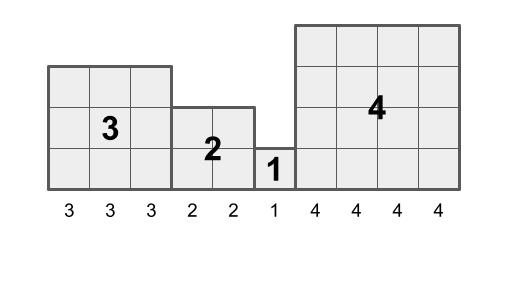시간 제한메모리 제한제출정답맞은 사람정답 비율
2 초 (추가 시간 없음) 1024 MB14612610488.889%

## 문제

Consider the histogram composed of $n$ squares with side lengths $a_1, a_2, \cdots, a_n$. Let's call the sequence $(a_1, a_2, \cdots, a_n)$ the histogram sequence of this histogram.

Let's consider the height of each column in this histogram. The first $a_1$ columns will each have height $a_1$, the following $a_2$ columns will each have height $a_2$, ... and the last $a_n$ columns will each have height $a_n$. Now, let us define the height sequence $(b_1, b_2, \cdots, b_{a_1 + a_2 + \cdots + a_n})$ where $b_j\ (1 \le j \le a_1+a_2+\cdots+a_n)$ is the height of the $j$-th column.

For example, the histogram with $(3, 2, 1, 4)$ as its histogram sequence has $(3, 3, 3, 2, 2, 1, 4, 4, 4, 4)$ as its height sequence.Write a program to find the histogram sequence given the height sequence.

## 입력

The first line contains a single integer $m\ (1 \le m \le 10^6)$ representing the length of the height sequence $\{b_i\}$ is given.

The second line of the input contains $m$ integers, the height sequence. Specifically, the $i$-th integer in the line is $b_i\ (1 \le b_i \le m)$.

The input is designed such that the provided height sequence corresponds to a valid histogram sequence.

## 출력

Output $n$ integers on a single line, $a_1, a_2, \cdots, a_n$ where $(a_1, a_2, \cdots, a_n)$ is the histogram sequence corresponding to the given height sequence. If there are multiple answers, any one of them will be accepted.

## 예제 입력 1

10
3 3 3 2 2 1 4 4 4 4


## 예제 출력 1

3 2 1 4


## 예제 입력 2

5
2 2 2 2 1


## 예제 출력 2

2 2 1